﻿ Transportation Algorithm with Volume Discount on Distribution Cost (A Case Study of the Nigerian Bottling Company Plc Owerri Plant)

### Transportation Algorithm with Volume Discount on Distribution Cost (A Case Study of the Nigerian Bot...

OSUJI GEORGE A., OGBONNA CHUKWUDI J., OPARA JUDEOPEN ACCESSPEER-REVIEWED

## Transportation Algorithm with Volume Discount on Distribution Cost (A Case Study of the Nigerian Bottling Company Plc Owerri Plant)

OSUJI GEORGE A.1, OGBONNA CHUKWUDI J.2, OPARA JUDE3,1Department of Statistics, Nnamdi Azikiwe University PMB 5025, Awka Anambra State Nigeria

2Department of Statistics, Federal University of Technology Owerri Nigeria PMB 1526, Owerri Nigeria

3Department of Statistics, Imo State University PMB 2000, Owerri Nigeria

### Abstract

This study is focused on the Application of Transportation Algorithm with volume Discount on distribution cost using Nigerian Bottling Company Plc Owerri Plant. This paper is intended to determine the quantity of Fanta (in crates), Coke (in crates) and Sprite (also in crates) that the Company should distribute in a month in order to minimize transportation cost and maximize profit. A problem of this nature was identified as a Nonlinear Transportation Problem (NTP), formulated in mathematical terms and solved by the Karush-Kuhn-Tucker (KKT) optimality condition for the NTP. A statistical software package was used to obtain the initial basic feasible solution using the Least Cost Method. Thus, analysis revealed that the optimal solution that gave minimum achievable cost of supply was the supply of 5000 crates of Fanta and 6000 crates of the same product to Umuahia market zone and Afikpo respectively. 7000 crates of Coke, 9000 crates and 1000 crates of the same product should be supplied to Orlu, Mbaise, and Afikpo market zones respectively. 6000, and 5000 crates of Sprite should be allocated to Mbaise and Umuahia market zones respectively, at a total cost of N377, 000.

• A., OSUJI GEORGE, OGBONNA CHUKWUDI J., and OPARA JUDE. "Transportation Algorithm with Volume Discount on Distribution Cost (A Case Study of the Nigerian Bottling Company Plc Owerri Plant)." American Journal of Applied Mathematics and Statistics 2.5 (2014): 318-323.
• A., O. G. , J., O. C. , & JUDE, O. (2014). Transportation Algorithm with Volume Discount on Distribution Cost (A Case Study of the Nigerian Bottling Company Plc Owerri Plant). American Journal of Applied Mathematics and Statistics, 2(5), 318-323.
• A., OSUJI GEORGE, OGBONNA CHUKWUDI J., and OPARA JUDE. "Transportation Algorithm with Volume Discount on Distribution Cost (A Case Study of the Nigerian Bottling Company Plc Owerri Plant)." American Journal of Applied Mathematics and Statistics 2, no. 5 (2014): 318-323.

 Import into BibTeX Import into EndNote Import into RefMan Import into RefWorks

### 1. Introduction

When considering transportation, various considerations are apparent. This consideration includes port selection, inland movement, and port to port carrier selection and delivery movement. In addition to these transportation concerns, distribution-related considerations must also be given attention, especially; packing/packaging, transit insurance, terms of sale, import duties, handling/loading and method of financing. Nevertheless, even freight companies projecting large volume movements can encounter serious transportation problem in organizing for distribution (Reep and Leaegood; 2002).

In the linear transportation problem (ordinary transportation problem) the cost per unit commodity shipped from a given source to a given destination is constant, regardless of the amount shipped. It is always supposed that the mileage (distance) from every source to every destination is fixed. To solve such transportation problem we have the streamlined simplex algorithm which is very efficient. However, in reality, we can see at least two cases that the transportation problem fails to be linear.

First, the cost per unit commodity transported may not be fixed for volume discounts sometimes are available for large shipments. This would make the cost function either piecewise linear or just separable concave function. In this case the problem may be formulated as piecewise linear or concave programming problem with linear constraints.

Second, in special conditions such as transporting emergency materials when natural calamity occurs or transporting military during war time, where carrying network may be destroyed, mileage from some sources to some destination are no longer definite. So the choice of different measures of distance leads to nonlinear (quadratic, convex, etc.) objective function.

In nonlinear transportation problem, its solution is more complex than that of linear transportation problem. In this work, solution procedures to the generalized transportation problem taking nonlinear cost function are investigated. In particular, the nonlinear transportation problem considered in this paper is stated as follows;

•  We are given a set of n sources of commodity with known supply capacity and a set of m destinations with known demands.

•  The function of transportation cost is nonlinear and differentiable for a unit of product from each source to each destination.

•  We are required to find the amount of product to be supplied from each source (may be market) to meet the demand of each destination in such a way as to minimize the total transportation cost.

Our approach to solve this problem is applying the existing general nonlinear programming algorithms to it making a suitable modification in order to use the special structure of the problem.

This paper seeks to solve a transportation problem with volume discount. The costs of goods are determined by factors such as: the costs of raw materials, labour, and transport. When cost of raw materials rises, so does the cost of the goods. Transportation cost also affects the pricing system. It is assumed that the cost of goods per unit shipped from a give source to a given destination is fixed regardless of the volume shipped. But in actuality the cost may not be fixed. Volume discounts are sometimes available for large shipments so that the marginal costs of shipping one unit might follow a particular pattern. Our focus will be to develop a mathematical model using optimization techniques to close the demand and supply gap by discounting so as to minimize total transportation cost. This research seeks to apply the existing general nonlinear programming algorithms to solve our problem.

### 2. Review of Related Literatures

Zangiabadi and Maleki (2007) presented a fuzzy goal programming approach to determine an optimal compromise solution for the multi-objective transportation problem by assuming that each objective function has a fuzzy goal. A special type of non-linear (hyperbolic) membership function is assigned to each objective function to describe each fuzzy goal. The approach focused on minimizing the negative deviation variables formed to obtain a compromise solution of the multi-objective transportation problem.

Lau et al. (2009) presented an algorithm called the fuzzy logic guided non-dominated sorting genetic algorithm to solve the multi-objective transportation problem that deals with the optimization of vehicle routing in which multiple depots, multiple customers, and multiple products were considered. Since the total traveling time is not always restrictive as a time constraint, the objective considered comprises not only the total traveling distance, but also the total traveling time. Lohgaonkar and Bajaj (2010) used fuzzy programming technique with linear and non-linear membership function (hyperbolic, exponential) to find the optimal compromise solution of a multi-objective capacitated transportation problem.

Caputo (2006) presented a methodology for optimally planning long-haul road transport activities through proper aggregation of customer orders in separate full-truckload or less-than-truckload shipment in order to minimize total transportation cost. He has demonstrated that evolutionary computation technique may be effective in tactical planning of transportation activities. The model shows that substantial savings on overall transportation cost may be achieved by adopting the methodology in a real life scenario.

Kikuchi (2000): Suggested that in many problems of transportation engineering and planning, the observed or derived values of the variables are approximate, yet the variables themselves must satisfy a set of rigid relationships dictated by physical principle. They proposed a simple adjustment method that finds the most appropriate set of crisp numbers. The method assumes that each observed value is an approximate number (or a fuzzy number) and the true value is found in the support of the membership function. This process was performed using the fuzzy linear programming method for each of many possible sets of values for the problem.

Frank (1970) developed an algorithm for reaching an optimal solution to the production-transportation problem for the convex case. The algorithm utilized the decomposition algorithm between a linear programming transportation problem which allocated previously set plant production quantities to various markets and a routine which optimally sets plant production quantities to equate total marginal production cost, including a shadow price representing a relative location cost determined from the transportation problem.

Shetty (1959) also formulated an algorithm to solve transportation problems taking nonlinear costs. He considered the case when a convex production cost is included at each supply center besides the linear transportation cost. Mohamed (1983) assessed the concavity of the cost curve brought about by economics of scale leads to multiple-optimality. The power of the simplex method in solving linear programs is based on the general theorem which states that the number of variables – including slack variables, whose values are positive in an optimal solution, is at most equal to the number of constraints in the problem. For this reason, nearsighted computational techniques are used to examine the corners of the feasible region (basic solution).

### 3. Methodology

3.1. The Karush-Kuhn-Tucker (Kkt) Optimality Condition for Nonlinear Programming Problem

Given the non linear programming problem (NNP):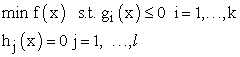(1)

3.1.1. Karush-Kuhn-Tucker Necessary Optimality Conditions

Theorem 1: Given the objective function f: ℝn → ℝ and the constraint function are gi: n → ℝ and hj: n → ℝ and I = {i: gi(x*) = 0}. In addition, suppose they are continuously differentiable at a feasible point x* and ∇gi(x*) for i ∈I and ∇hj(x*) for j = 1, …, l be linearly independent. If x* is minimizer of the problem (NPP), then there exist scalars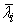; i = 1, …, k and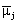; j =1, …, l, called Lagrange multipliers, such that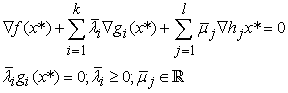(2)

3.1.2. Karush-Kuhn-Tucker Sufficient Optimality Conditions for Convex Npp

Further, if f and each gi are convex, each hj is affine, then the above necessary optimality conditions will be also sufficient (Simons; 2006).

Justification

Let x be any feasible point different form x*. From the first KKT conditions we obtain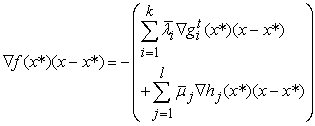Since each gi(x) is convex,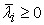and ∇hj(x*)(x – x*) = 0 ∀j, we also have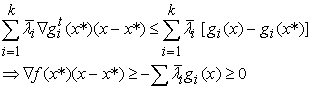From convexity of f(x), therefore, we get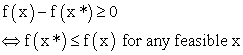### 4. Solution Procedures to the Nonlinear Transportation Problem (Ntp)

In this section, we consider a transportation problem with nonlinear cost function. We try to find different solution procedures depending on the nature of the objective function. Before going to the different special cases, let’s formulate the KKT condition and general algorithm for the problem.

Given a differentiable function C: ℝnm → ℝ.

We consider a nonlinear transportation problem (NTP)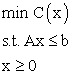(3)

where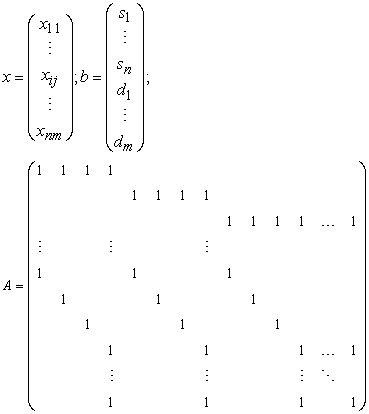The KKT Optimality Condition for the NTP

The transportation table is given as: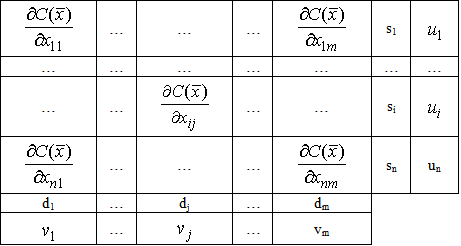where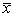is the current basic solution.

The Lagrange function for the NTP is formulated as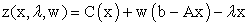(4)

where λ and w are Lagrange multipliers andThe optimal pointshould satisfy the KKT conditions: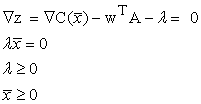Specifically for each cell (i, j) we have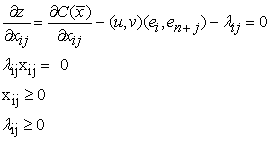(5)

Where k = 1 … nm and w = (u, v) = (u1 u2… un, v1… vm), ek∈ℝm+n is a vector of zeros except at position k which is 1.

From the conditions (5) and λk ≥ 0, we get,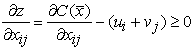(6)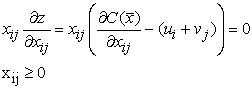(7)

General solution procedure for the NTP.

Initialization

Find an initial basic feasible solution.

Iteration

Step I: ifis KKT point, stop. Otherwise go to the next step.

Step II: Find the new feasible solution that improves the cost function and go to step 1(Kidist; 2007).

### 5. Transportation Problem With Concave Cost Functions

For large distributions, volume discount may be available sometimes. In this case the cost function of the transportation problem generally takes concave structure for it is separable and the marginal cost (cost per unit commodity distributed) decreases with increase in the amount of distribution; because of the total cost increase per addition of unit commodity distributed. The discount

1. May be either directly related to the unit commodity.

2. Or have the same rate for some amount.

Case 1: If the discount is directly related to the unit commodity, the resulting cost function will be continuous and have continuous first partial derivatives.

Nonlinear programming formulation of such a problem is given by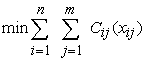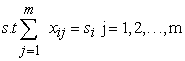(8)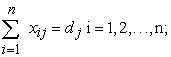Where Cij: ℝ→ℝ.

### 6. The Transportation Concave Simplex Algorithm (Tcs)

Initialization

Find the initial basic feasible solution using some rule like west corner rule.

Iteration

Step 1: Determine the values of ui and vi from the equation,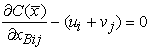(9)

Where xBij are the basic variables.

Step 2: If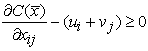(10)

for all xij – non basic, stop,is KKT point. Otherwise go to step 3.

Step 3: Calculate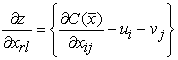(11)

xrl will enter the basis. Allocate xrl = θ where θ is found as in the linear transportation case.

Adjust the allocations so that the constraints are satisfied.

Determine the leaving variable say xBrk, where xBrk is the basic variable which comes to zero first while making the adjustment. Then find the new basic variables and go to step 1.

### 7. Data Analysis

The Nigerian Bottling Company Plc Owerri Plant, a distributor of various kinds of drinks located in ; sell the same product to different market segments within the neighborhood. For the sake of this work, only five market segments (Mbaise, Orlu, , Umuahia, and Afikpo) and three soft drinks (Fanta, Coke and Sprite) shall be taken for this study. The cost of purchasing and transporting the drinks from the traders place to the market centres is given in Table 1 below.

#### Table 1. Cost of Transporting the Drinks to the various market zones

All the value in the Table 1 apart from requirements and supply are in Nigerian Currency (Naira) value. The Policy of the Company assumes discounts on each product transported from source to destination and it is directly related to the unit commodity purchased and transported, and the percentage discounts are shown in Table 2.

The problem is to determine how many creates of each product to be transported from the source to each destination on a monthly basis in order to minimize the total transportation cost.

#### Table 3. Forming the transportation tableau

To form the transportation tableau, let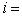product to be supplied;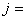destination of each product;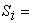the capacity of source node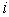,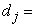the demand of destination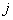;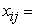the total capacity from sourceto destination;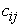= the per unit of transporting commodity fromto destination. If we suppose that discount is given on each crate transported fromto, then the non-linear transportation problem can be formulated as

Minimize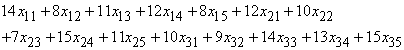Subject to: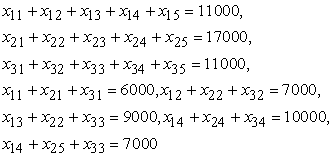Where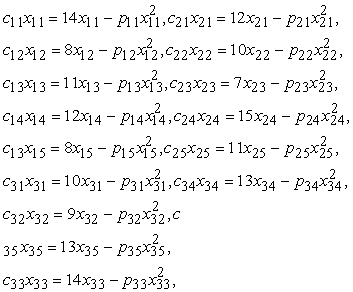If we allow the following discounts on each transported product I from the source to each of the destinations, we obtain the cost functionwhich can be expressed as;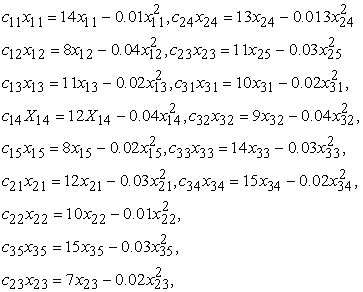The tableau is then developed as below;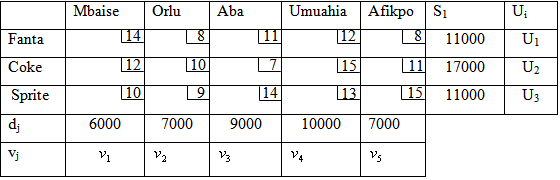Using the least cost method, we get the initial basic solution as shown below.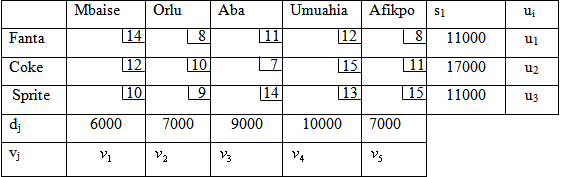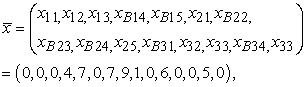in thousand with the total transportation cost of N377,000.

Now, we use the KKT optimality conditions to improve upon our solution. The partial derivatives atfor the cost function are given as;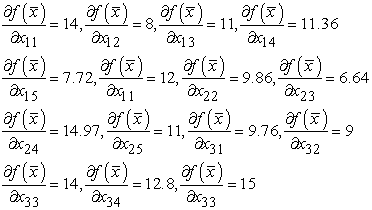Now we find from the cost equation of the occupied cell;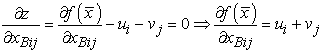Thus,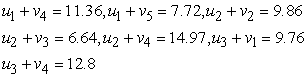Letting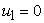, from the equation above, we obtain;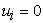,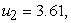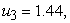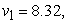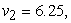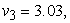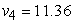and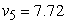.

We proceed to find the net evaluation factor or the reduced costs for the non-basic variable.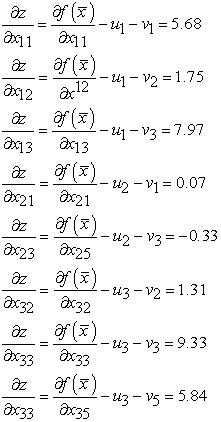The presence of a negative value for the reduced cost signifies non optimality; hence we readjust. It is obvious that from the above, the minimum reduced costs for the non-basic variable is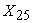. Thereforeshould enter the basis since it is the only negative reduced cost.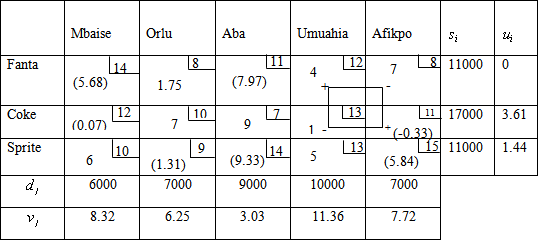The basic variable with the least value among the corners having signed in the loop is the leaving variable. Hence,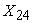with the least value of 1 is the leaving variable. Thus, we increase the corners with + sign by 1, reduce the ones with – sign by 1. The adjusted tableau becomes: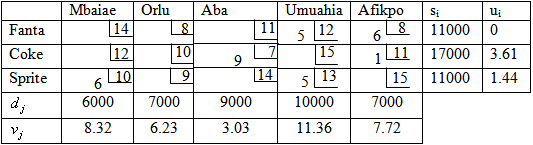The reduced costs for the non-basic ones at a basic feasible point,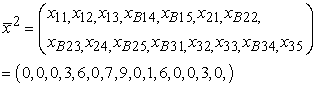Will be;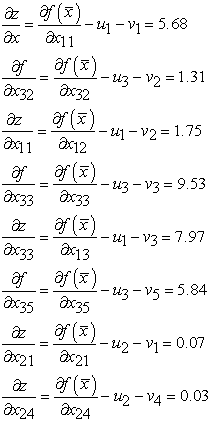Since all the reduced costs for the non-basic variables are all positive, it implies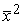is the KKT optimality point. Since optimal solution is our primary goal, we then proceed to make our allocation and calculate our total optimal cost of transportation. Hence, the feasible solution that 5000 crates of Fanta and 6000 crates of the same product should be supplied to Umuahia market zone and Afikpo respectively. 7000 crates of Coke, 9000 crates and 1000 crates of the same product should be supplied to Orlu, Mbaise, and Afikpo market zones respectively. 6000, and 5000 crates of Sprite should be allocated to Mbaise and Umuahia market zones respectively. Total cost = 5000 (12) + 6000(8) + 7000(10) + 9000(7) +1000(11) + 6000 (10) + 5000(13) = N377, 000.

### 8. Conclusion

We have described the transportation problem of Nigerian Bottling Company Plc Owerri Plant as a non-linear transportation problem. We also applied KKT optimality algorithm to solve the company’s problem. Note that our research focused on the model of the non-linear transportation problem for a particular company in . It can however be applied to any situation that can be modeled as such.

This paper aimed at solving transportation problem with volume discount on quantity of goods shipped which is a non-linear transportation problem. Using KKT optimality algorithm, with a set of data from a Nigerian company, it was observed that the optimal solution that gave minimum achievable cost of supply was the supply of 5000 crates of Fanta and 6000 crates of the same product to Umuahia market zone and Afikpo respectively. 7000 crates of Coke, 9000 crates and 1000 crates of the same product should be supplied to Orlu, Mbaise, and Afikpo market zones respectively. 6000, and 5000 crates of Sprite should be allocated to Mbaise and Umuahia market zones respectively, at a cost of N377, 000.

Using the more scientific transportation problem model for the company’s transportation problem gave a better result. Management may benefit from the proposed approach for their transportation problem purposes. We therefore recommend that the transportation problem model should be adopted by the company for their transportation problem planning.

### References

  Caputo, A.C. (2006). A genetic approach for freight transportation planning, Industrial Management and Data Systems, Vol. 106 No. 5.In article CrossRef  Frank, S. J. (1970). A Decomposition Algorithm for multifacility production transportation problem with nonlinear production costs, Econometrics, Vol. 38 No. 3.In article  Kidist, T. (2007). Nonlinear Transportation Problems. A paper submitted to the department of mathematics of Addis Asaba University.In article  Kikuchi, S.A. (2000). A method to defuzzify the number: transportation problem application, Fuzzy Sets and Systems, vol. 116.In article CrossRef  Lau, H.C.W.; Chan, T.M.; Tsui, W.T.; Chan, F.T.S.; HO, G.T.S and Choy K.L. (2009). A fuzzy guided multi-objective evolutionary algorithm model for solving Transportation problem. Expert System with Applications: An International Journal. Vol. 36.In article  Lohgaonkar, M.H. and Bajaj, V.H. (2010). Fuzzy approach to solve multi-objective capacitated transportation problem, International Journal of Bioinformatics Research. Vol. 2.In article  Reep, J. and Leaengood S. (June 2002). Transportation problem: A Special Case of Linear Programming Problems, Operations Research Society of America.In article  Shetty, C.M. (1959). A Solution to the Transportation Problem with Nonlinear Costs," Operation Research. Vol. 7. No. 5.In article  Simons, A.R. (2006). Nonlinear Programming for Operation research, Society for Industrial and Applied Mathematics, Vol. 10, No. 1.In article  Mohamed, A. (1983). An iterative procedure for solving the incapacitated production distribution problem under concave cost function, International Journal of operations and management, Vol. 16. No. 3.In article  Zangiabadi, M. and Maleki, H.R. (2007). Fuzzy goal programming for multi-objective transportation problems. Applied Mathematics and Computation, vol. 24, pp. 449-460.In article